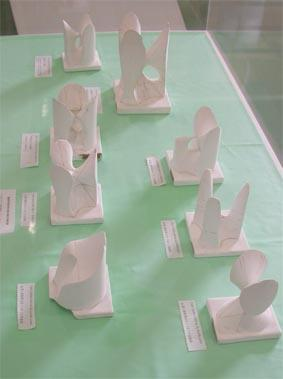## 特異点をもつ3次曲面

Cubics with SingularitiesThese are cubic surfaces with singularities called rational double points. These singularities are described by the extended Dynkin digaram of type $E_6$.

Cubic with three $A_1$ double points

Cubic with an $A_2$ double point

Cubic with a $D_4$ double point

Cubic with a $D_5$ double point